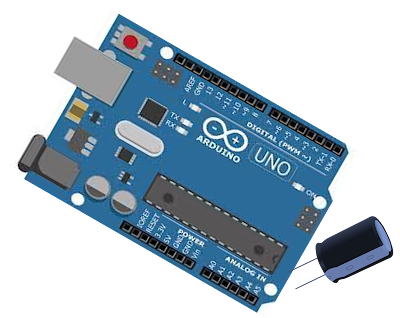## Posts

Showing posts with the label Arduino simulation - Capacitance meter

### Arduino simulation - Capacitance meterThis video tutorial illustrates the Arduino based simulation of capacitance meter / capacitance measurement of capacitors & supercapacitors with Tinkercad simulation.   Principle for this measurement is explained in the following page: https://www.enote.page/2022/01/capacitor-charging-discharging-time.html   Estimation of capacitance of capacitors & supercapacitors by simulating charging & discharging is explained in the following page: https://www.enote.page/2021/06/capacitor-simulation.html   Components required Name Number Component C1 1 100 nF to 10 mF Capacitor R1 1 10 kΩ Resistor U1 1 Arduino Uno R3 board Meter2 1 Voltage meter R2 1 220 Ω Resistor     Increase the resistance value from 10K, if the charging time is too short. Decrease the